# Model Diagram of Synchronous Motor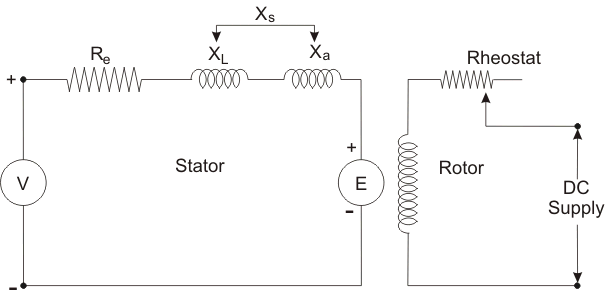V = Terminal voltage
Re = Effective resistance
XL = Leakage reactance
Xa = Fictious reactance
Xs = Synchronous reactance
E = Counter emf

In case of a synchronous motor, revolving field structure is to be energized by direct current (DC current). In stator winding two effects are to be considered the effect of field cutting stator conductors at synchronous speed and the effect of stator revolving field. A voltage induced in the stator winding due to the rotating magnetic field.

This voltage is called counter emf (E) oppose the applied voltage (V) to the stator. The magnitude of induced emf depends upon strength of excitation current. In the stator section there have two reactance counted – one is leakage reactance and the other is fictitious reactance.

The effect of armature reaction can be substituted by fictitious reactance (Xa) which when combined with the leakage reactance of the armature gives synchronous reactance (Xs) combined with the armature effective resistance (Re) gives the synchronous impedence (Zs).

## Zero Power Factor Method or Potier Triangle

Before discussing the Potier triangle we have to discuss Potier characteristic. The Zero-power factor characteristic (ZPFC) of an alternator is a curve of the armature terminal voltage per phase plotted against the field current with constant rated armature current at synchronous speed and zero lagging power factor (p.f.).

For maintaining very low p.f. (zero) alternator is loaded by an under excited synchronous motor. The shape of zero power factor characteristic graph is very much like of the O.C.C. displaced downward horizontally.

The Phasor diagram as follows –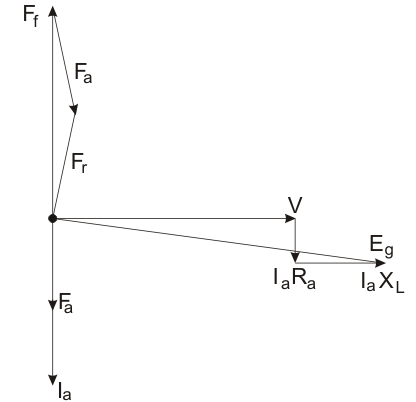Here,
Y = Terminal voltage
Ia = Armature current
Ra = Armature resistance
XL = Leakage reactance
Eg = Generated voltage per phase
Fa = Armature reaction mmf
Ff = Field mmf
Fr = Resultant emf
If we neglect the armature resistance the Phasor will as follows-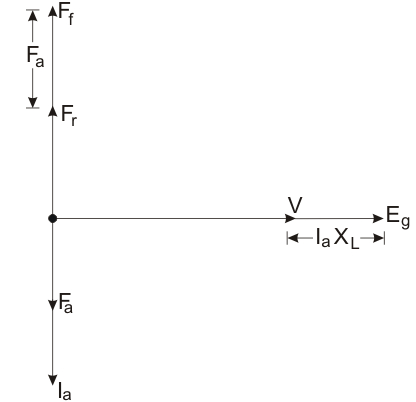Taking the reference terminal voltage at zero p.f. lagging, the armature current lag behind voltage by 90o. Here IaRa parallel to Ia, IaXL perpendicular to Ia.
Then we can say that from first phasor diagram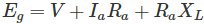From the second phasor diagram we can say that terminal voltage (V), the reactance voltage drop (IaXL), and the generated voltage (Eg) are in phase.
The arithmetically we say that :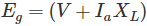Also the three mmf phasor are in phase so that we can say that :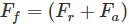If we convert this equation into the equivalent field current by dividing its both sides by Tf which is the effective number of turns per pole on the roter field.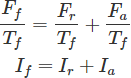Where,
If = Field current
Ir = Resultant current
Ia = Armature current

Let consider ‘b’ at zero p.f. carve at rated terminal voltage (v) and field current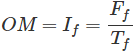The armature current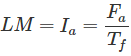Resultant current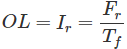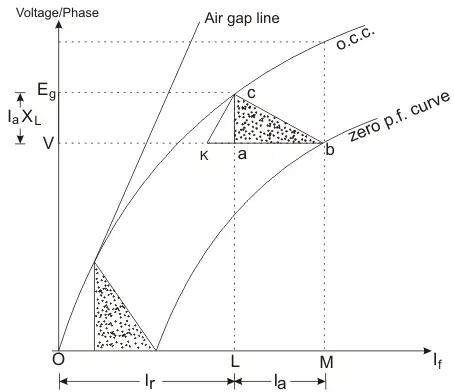The field current OL would result in generated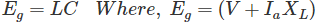So that the vertical distance AC must be equal to leakage – reactance voltage drop (IaXL)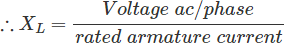The triangle formed by the vertices a, b, c called Potier Triangle.

Want To Learn Faster? 🎓
Get electrical articles delivered to your inbox every week.
No credit card required—it’s 100% free.